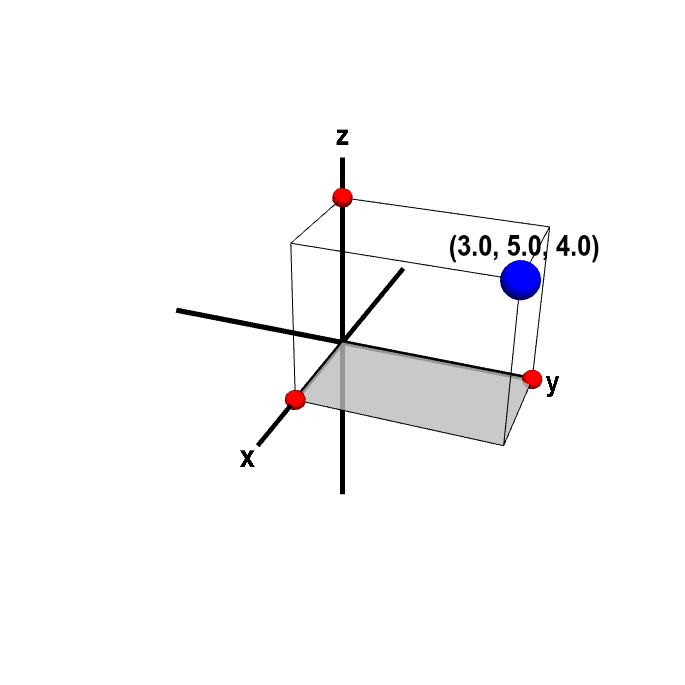# Math Insight

### Applet: Cartesian coordinates of a point in three dimensionsThe Cartesian coordinates $(x,y,z)$ of a point in three-dimensions specify the signed distance from the origin along the $x$, $y$, and $z$-axes, respectively. The rectangular box has opposite corners at the origin and at the blue point. The three coordinates of the blue point are represented by the red points, which are the corners of the box along each axis. You can change the point by dragging the blue point with the mouse. Alternatively, you can independently change one of the coordinates by dragging a red point.